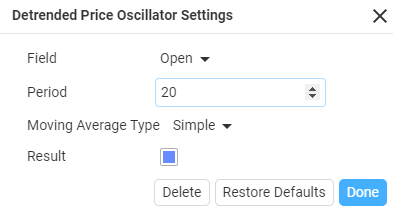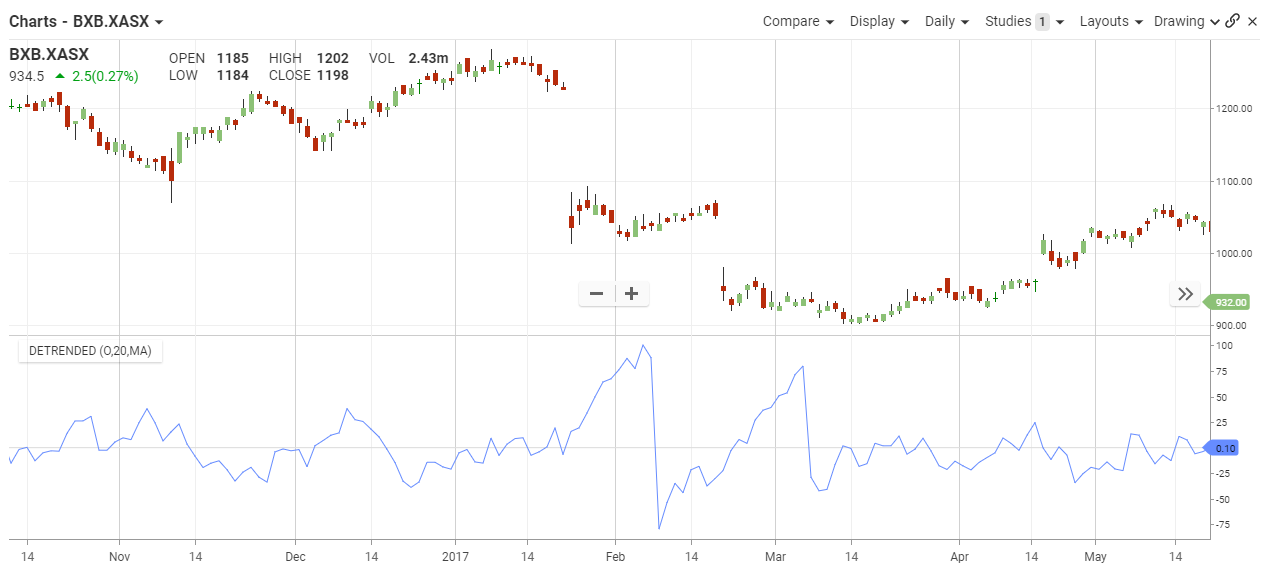## Detrended Price Oscillator

#### The Basics

The Detrended Price Oscillator (DPO) is an indicator designed to remove trend from price and make it easier to identify cycles. DPO does not extend to the last date because it is based on a displaced moving average. However, alignment with the most recent is not an issue because DPO is not a momentum oscillator. Instead, DPO is used to identify cycles highs/lows and estimate cycle length.

Indicator Type

Oscillator

Markets

All cash and futures, not options

Works Best

Identifying Cycles markets

Formula

Calculation: Price (X/2 + 1) periods ago minus X-period simple moving average

Where X is the number of periods; 20 or 30 periods is common.

Parameters#### Theory

The Detrended Price Oscillator (DPO) measures the difference between a past price and a moving average. Keep in mind that DPO is itself displaced to the left. The indicator oscillates above/below zero as prices move above/below the displaced moving average.

#### InterpretationThe Detrended Price Oscillator shows the difference between a past price and a simple moving average. In contrast to other price oscillators, DPO is not a momentum indicator. Instead, it is simply designed to identify cycles with its peaks and troughs. Cycles can be estimated by counting the periods between peaks or troughs. Users can experiment with shorter and longer DPO settings to find the best fit.

#### Maths

Price {X/2 + 1} periods ago less the X-period simple moving average.

X refers to the number of periods used to calculate the Detrended Price Oscillator. A 20-day DPO would use a 20-day SMA that is displaced by 11 periods {20/2 + 1 = 11}. This displacement shifts the 20-day SMA 11 days to the left, which puts it in the middle of the look-back period. The value of the 20-day SMA is then subtracted from the price in the middle of this look-back period. In short, DPO(20) equals price 11 days ago less the 20-day SMA.